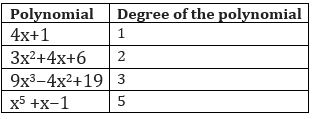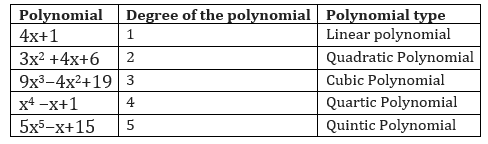# What is a Polynomial expression

Welcome to Class 9 Maths chapter 2 Polynomials notes.The topics in this page are what is Polynomial expression, how to find the degree of a polynomial explanations, Value of the polynomial and Zeros or roots of the polynomial along with examples, and questions and answers .This is according to CBSE and the NCERT textbook. If you like the study material, feel free to share the link as much as possible.

Table of Content

## Constants and Variable

• The value of constant remains same throughout the case and it does not changes in the given situation.
• They are generally denoted by $a,b,c$ etc.
• Variable value keep changing and they are generally denoted by the letters $x,y,z$ etc.

## What is a Polynomial expression

A polynomial expression S(x) in one variable x is an algebraic expression in x term as
$S(x)=a_nx^n+a_{n-1}x^{n-1}+a_{n-2}x^{n-2}+.....+a_1x+a_0$
Where $a_n,a_{n-1},....,a_1,a_0$ are constant and real numbers and an is not equal to zero

Some important points to remember
• an,an-1,...,a,a0 are called the coefficients for xn,xn-1 ,..,x,x0
• n is called the degree of the polynomial
• when $a_n,a_{n-1},....,a_1,a_0$ are zero, it is called zero polynomial
• A constant polynomial is the polynomial with zero degree, it is a constant value polynomial
• A polynomial of one item is called monomial, two items binomial and three items as trinomial
• A polynomial of one degree is called linear polynomial, two degree as quadratic polynomial and degree three as cubic polynomial

We can also have polynomials in more than one variable. For example, $x^2 + y^2 +z^2$ (where variables are $x, y \; and \; z$) is a polynomial in three variables. Similarly $p^2 + q^4 + r$ where the variables are $p, q \; and \; r$) is polynomials in three variables

Also if a0 = a1 = a2 = a3 = . . . = an = 0 (all the constants are zero), we get the zero polynomial, which is denoted by 0. Now we may ask What is the degree of the zero polynomial? The degree of the zero polynomial is not defined

## how to find the degree of a polynomial

The degree of a polynomial is the greatest exponent of the variable in the polynomial when the polyomial is expression in its canonical form consisting of a linear combination of monomials.
The degree of a term is the sum of the exponents of the variables that appear in it
Lets take a example of polynomial
$S(x) =x^3 +x^2+ 5$
Here the highest exponent of x is 3. So degree of the polynomial is 3

SimilarlyWe have polynomial types depending on degree alsoFor constant polynomial
$P(x) = 9$
It can be expression as
$P(x) =9x^0$

So degree is zero

## Value of the polynomial:

Lets take a example of polynomial
$S(x) =x^2 +1$
Then
When we put the value of x=2,then
$S(2)=4+1=5$
The S(2) is the called the value of polynomial at x=2
In General terms, the value of polynomial at x=a is S(a)

## Zero's or roots of the polynomial

It is a solution to the polynomial equation $S(x)=0$ i.e. a number "a" is said to be a zero of a polynomial if S(a) = 0.
If we draw the graph of S(x) =0, the values where the curve cuts the X-axis are called Zeros of the polynomial
a. Linear polynomial has only one root
b. A zero polynomial has all the real number as roots
c. A constant polynomial has no zeros
d. A zero of polynomial need not to be 0

Check out the below video for Polynomial

### Quiz Time

Question 1 Value of $P(x)=x^2 +16+10x$ at x=-1 is ?
A. 7
B. -7
C. 1
D. 0
Question 2 Degree of the polynomial $x^{3} + 13 x^{2} - 41 x + 45$ is ?
A. 2
B. 3
C. 4
D. 1
Question 3 Which one of these is a binomial
A. $(x^{10}+1)$
B. $x^{2}$
C. $(x^{10}+x+11)$
D. $(x^{10}+x^9 +1)$
Question 4Which of these is a quadratic polynomial
A. $x^{3} + 13 x^{2} + 45$
B. $x^{4} + 12 x^{2} + 41 x + 45$
C. $x^{3} + 13 x^{2} + 41 x$
D.None of the above
Question 5 which of these is a zero polynomial
A. 0
B. 1
C. $4x^0$
D. none of the above
Question 6 if $P(x) =x^2 -1$, The number of zeros are ?
A. 1
B. 2
C. 3
D. 0